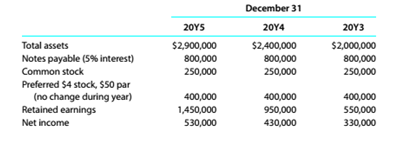Chapter 9, Problem 9.17E

Chapter
Section
Textbook Problem

Profitability metricsThe following selected data were taken from the financial statements of The O'Malley Group Inc. for December 31, 20Y5. 20Y4. and 20Y3:No dividends on common stock were declared between 20Y3 and 20Y5.a.Determine the return on total assets, the return on stockholders' equity, and the return on common stockholders' equity Tor the years 20Y4 and 20Y5. Round to one decimal place. b. What conclusions can be drawn from these data as to the company's profitability?

To determine

(a)

Concept Introduction:

Profitability analysis:

Ithelps to evaluate the ability of a company to generate income relative to revenue, assets, operating cost and shareholders' equity during a specific period.

It measures how efficiently the resources have been utilised to generate profit.

To calculate:

Return on total assets, return on common stockholders' equity and return on stockholders' equity for the year 20Y4 and 20Y5

Explanation

Return on total assets=net income+interest expenses Average total assets

Return on shareholders'equity=net income-preferred dividends shareholders equity

Return on commonstock holders equity =net income common stock holders equity

 Particulars 20Y5 20Y4 Net income $530,000$430,000 Interest on Notes Payable $800,000×5%=$40,000 $800,000×5%=$40,000 Preferred Dividends $30,000$30,000
 Particulars 20Y5 20Y4 Common Stock (A) $250,000$250,000 Preferred Stock (B) $400,000$400,000 Retained Earnings (C) $1,450,000$950,000 Total Stockholders' Equity (A+B+C) $2,100,000$1,600,000 Total Common Stockholders' Equity (A+C) $1,700,000$1,200,000 Total Assets (Beginning) $2,400,000$2,000,000 Total Assets (Ending) $2,900,000$2,400,000 Total Assets (Average) $2,900,000+$2,400,0002 =$2,650,000$2
To determine

(b)

Concept Introduction:

Profitability analysis:

It is used to evaluate the ability of a company to generate income relative to revenue, assets, operating cost and shareholders' equity during a specific period.

It measures how efficiently the resources have been utilised to generate profit.

To analyze:

The calculated ratios to evaluate company's profitability.

Still sussing out bartleby?

Check out a sample textbook solution.

See a sample solution

The Solution to Your Study Problems

Bartleby provides explanations to thousands of textbook problems written by our experts, many with advanced degrees!

Get Started

Find more solutions based on key concepts